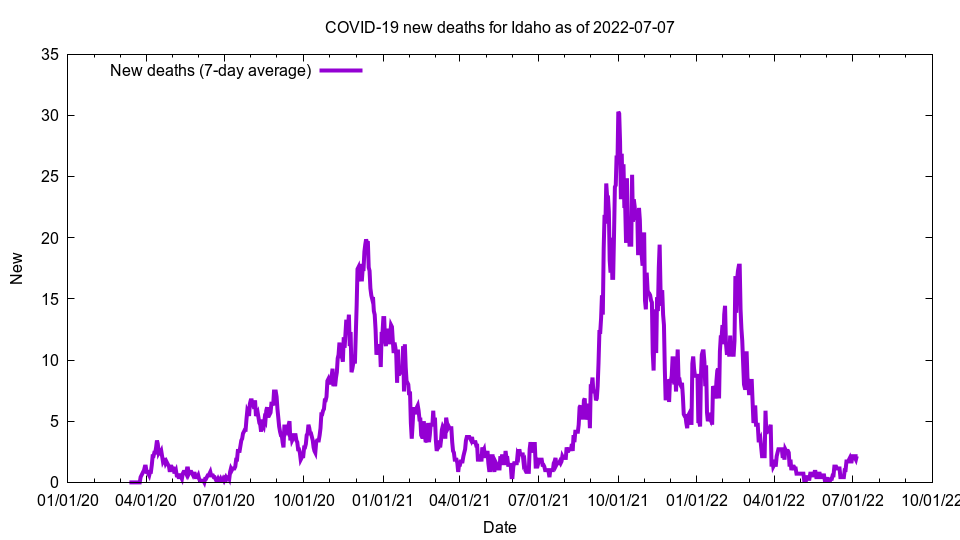Go to: USA - Top - Site map

This is a graph showing COVID-19 deaths.

# IdahoThis image shows doubling time for deaths for Idaho as of 2020-09-24

Deaths: 458
Deaths Per 100,000: 25.63
Deaths 14-day per 100k: 2.85
New deaths (7-day average): 3.29
Growth: 0.74%
Doubling days (calculated): 94.13
Doubling days (actual): 49.00

The above graph shows doubling time, i.e. the number of days it takes for deaths to double. The purple line is calculated doubling time: The number of days, based on 7-day average growth, for cases to double. The green line is actual doubling time: How many days ago did we have half the number of cases. In both cases, the higher the line, the slower the COVID-19 growth.

Deaths is total COVID-19 deaths; deaths per 100,000 is deaths per 100,000 people; deaths 14-day per 100k is the total number of deaths over the last 14 days, added together, then multiplied by 100,000, then divided by the population. New deaths is the number of deaths we have had per day on average over the last seven days. Growth is the increase in deaths compared to the previous number of deaths; the number is a 7-day average. Doubling days is the number of days it will take for deaths to double at the current growth rate (calculated), or the number of days in the past we have had half the current number of deaths (actual).

The data comes from The New York Times and the code to generate these pages is open source and available on GitHub. The number of actual COVID-19 cases and deaths are almost certainly higher than these figures.